Function Repository Resource:

# NewtonMethodPlot

Plot the function together with a graphical display of the Newton iterations approximating its root

Contributed by: Dennis M Schneider
 ResourceFunction["NewtonMethodPlot"][f,{x,xmin,xmax},pt] returns a plot of f from x=xmin to x=xmax, together with illustrations representing the iterations of Newton’s root-finding method, starting at x=pt.

## Details and Options

Starting with an initial guess x0 for the zero of the function, the next approximation x1 is the horizontal intercept of the tangent line at x0. The process is repeated until a sufficiently accurate value is obtained.
The following options are accepted:
 "DrawGraph" True whether to include the graph "Iterations" 5 the number of iterations to perform "LineStyle" Thick graphics directive to specify the style for the line "PointStyle" PointSize[0.016] graphics directive to specify the style for the point "PrintDisplay" True whether to include the table of iteration values WorkingPrecision MachinePrecision determines the number of digits used in internal calculations

## Examples

### Basic Examples (4)

Illustrate Newton’s method on a simple function:

 In:=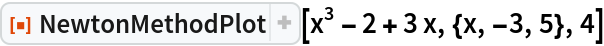Out=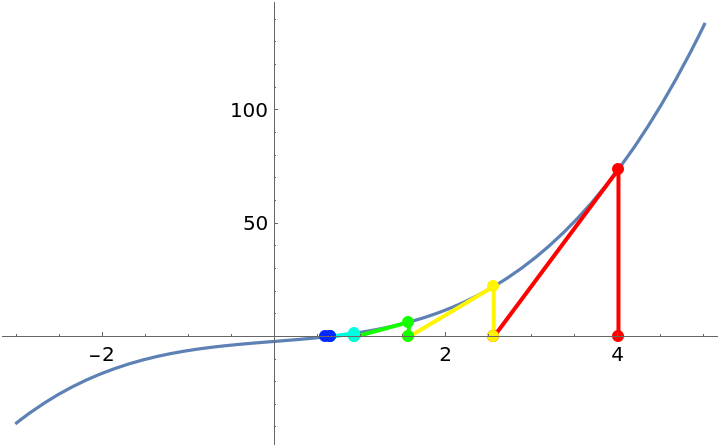Increase the number of iterations:

 In:=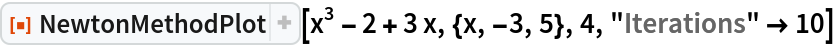Out=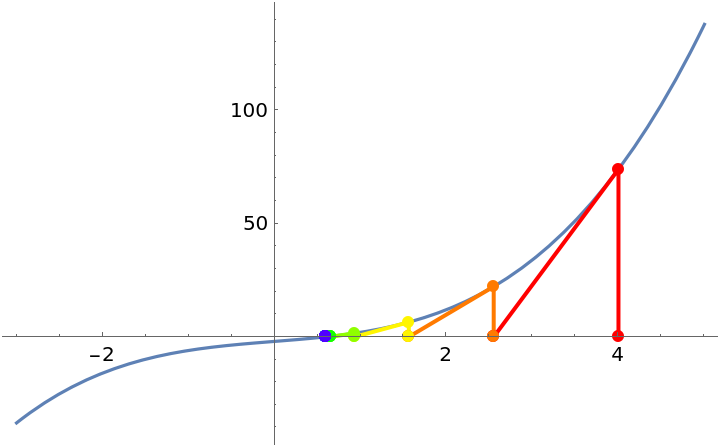Newton’s method can cycle:

 In:=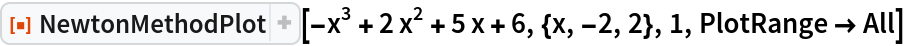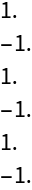Out=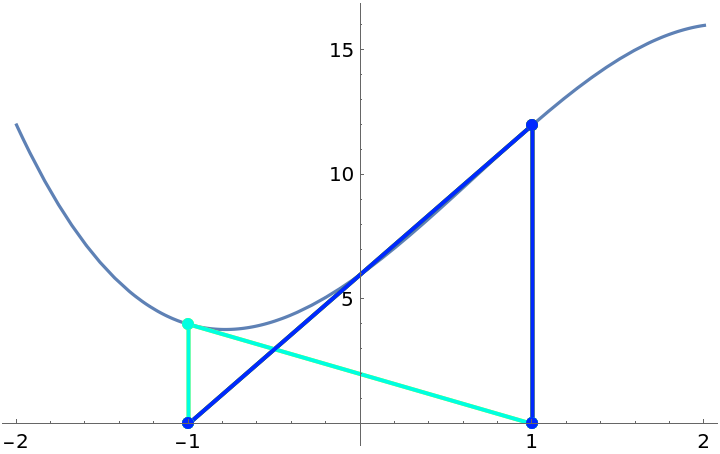Newton’s method may "converge" to a cycle:

 In:=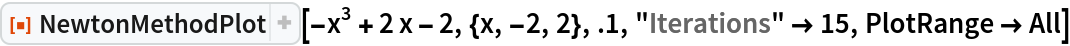Out=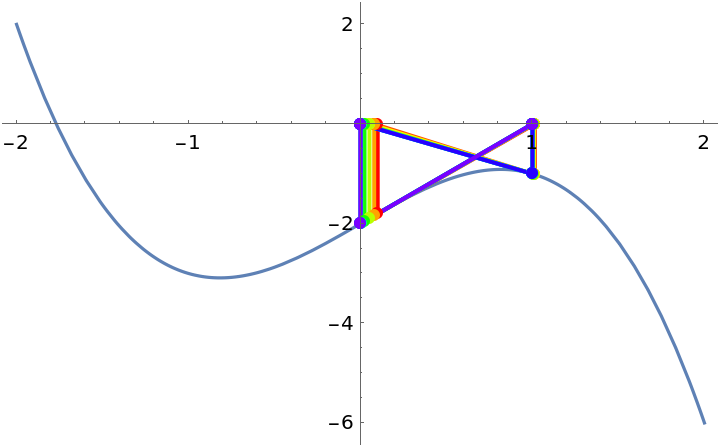### Possible Issues (2)

Use Surd when necessary:

 In:=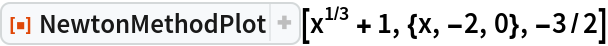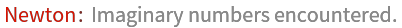In:=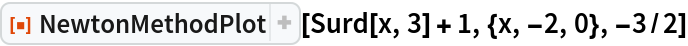Out=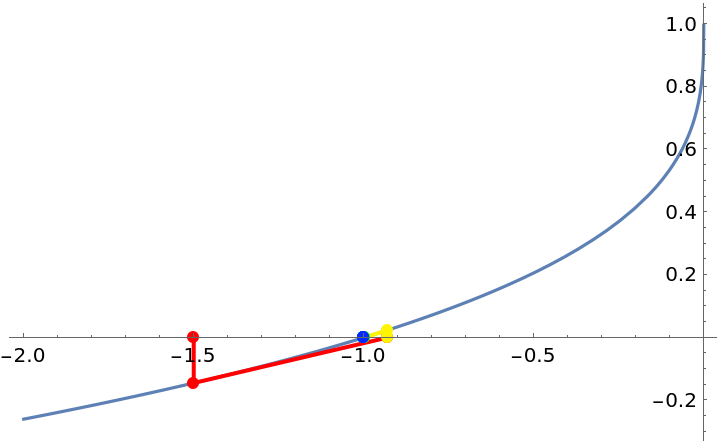Three examples that show that Newton’s method may converge to an unexpected root when it begins or lands near a zero of the derivative:

 In:=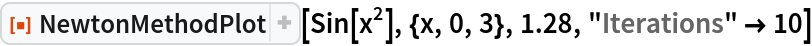Out=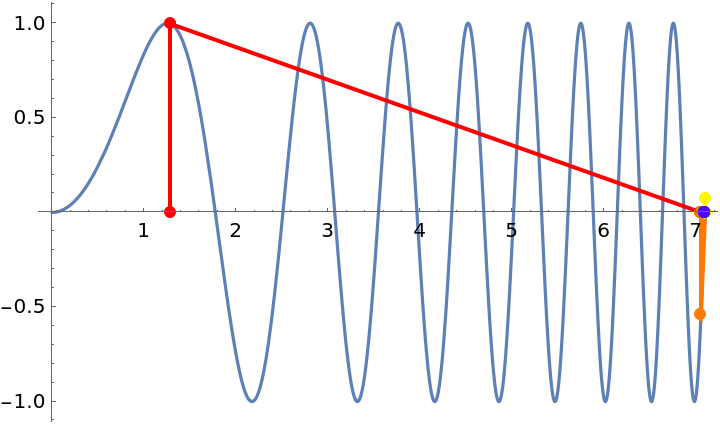In:=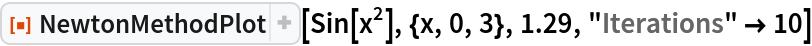Out=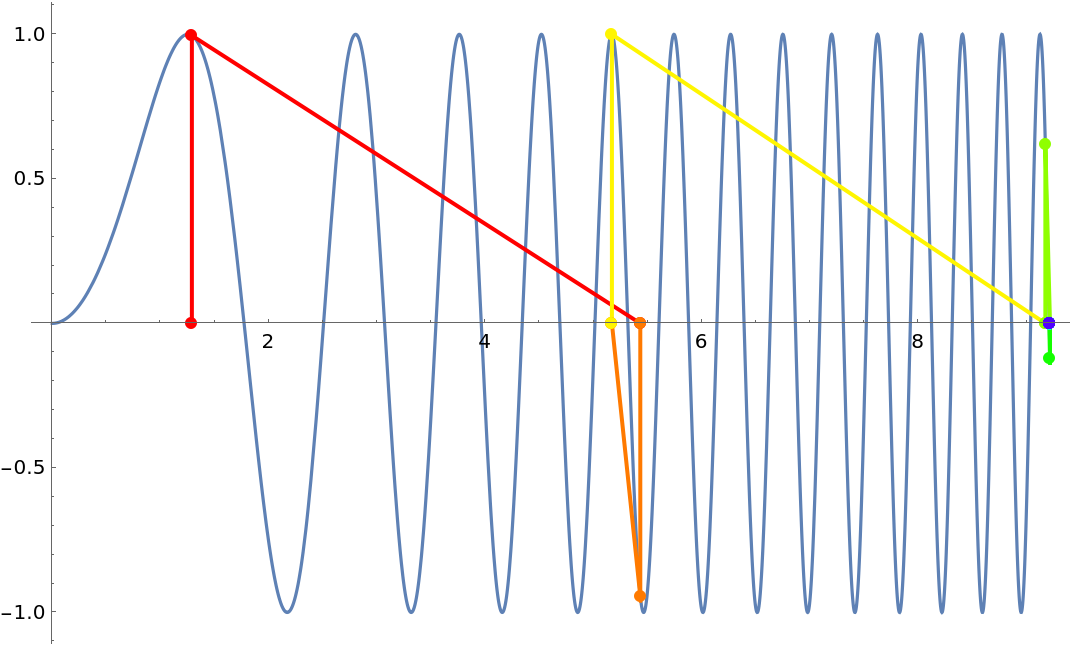In:=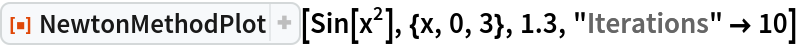Out=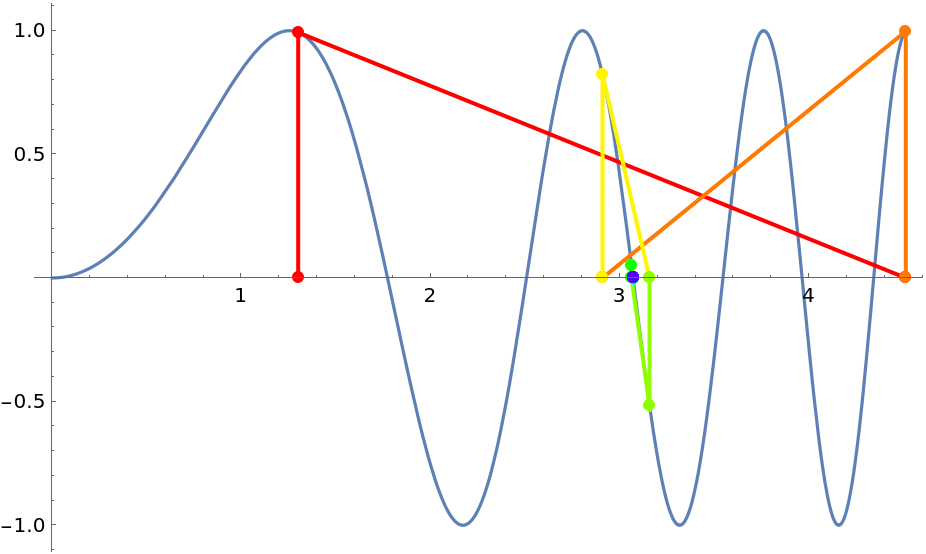## Publisher

Dennis M Schneider

## Version History

• 1.0.0 – 20 August 2019